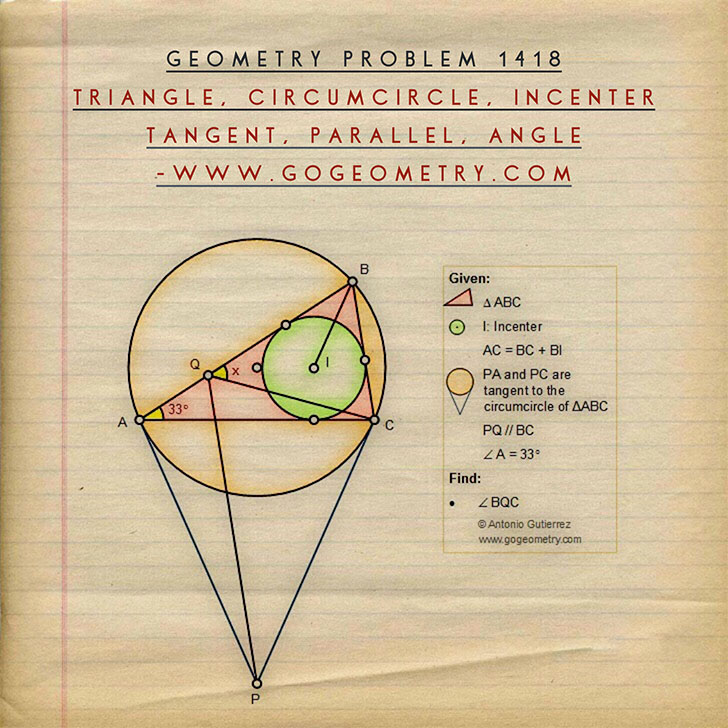# Geometry Problem 1418: Triangle, Circumcircle, Incenter, Side, Sum of Segments, Tangent, Parallel, Angle

### Proposition

Triangle ABC is such that angle A = 35 degree and AC = BC + BI where I is the incenter of the triangle. Tangents at A and C to the circumcircle of triangle ABC meet in P and PQ is drawn parallel to BC to meet AB at Q. Find the measure of the angle BQC.

This entry contributed by Ajit Athle.### Poster of Problem 1418: Sketching, iPad, Typography, Art